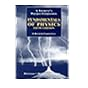Masinde Muliro University of Science and Technology Library CatalogueNormal view

# Fundamentals of physics / [David] Halliday & [Robert] Resnick, Jearl Walker.

Material type:TextPublisher: Hoboken, NJ : Wiley, c2003Edition: 6th edDescription: xxvi, 1144 p. : ill. (some col.), col. map ; 29 cmISBN: 9780470469088Other title: Halliday & Resnick fundamentals of physicsDDC classification: 530 LOC classification: QC21.2 | .R3 2003
Contents:
Measurement -- Motion along a straight line -- Vectors -- Motion in two and three dimensions -- Force and motion I -- Force and motion II -- Kinetic energy and work -- Potential energy and conservation of energy -- Center of mass and linear momentum -- Rotation -- Rolling, torque, and angular momentum -- Equilibrium and elasticity -- Gravitation -- Fluids -- Oscillations -- Waves I -- Waves II -- Temperature, heat, and the first law of thermodynamics -- The kinetic theory of gases -- Entropy and the second law of thermodynamics -- Electric charge -- Electric fields -- Gauss' law -- Electric potential -- Capacitance -- Current and resistance -- Circuits -- Magnetic fields -- Magnetic fields due to currents -- Induction and inductance -- Electromagnetic oscillations and alternating current -- Maxwell's equations; magnetism of matter -- Electromagnetic waves -- Images -- Interference -- Diffraction -- Relativity -- Photons and matter waves -- More about matter waves -- All about atoms -- Conduction of electricity in solids -- Nuclear physics -- Energy from the nucleus -- Quarks, leptons, and the big bang.
List(s) this item appears in: ELECTRICITY AND MAGNETISM | WAVES AND VIBRATION | THERMODYNAMICS
Tags from this library: No tags from this library for this title.Average rating: 0.0 (0 votes)
Item type Current location Call number Status Date due BarcodeBook (Long Loan)
General Stacks
QC21.2 .R3 2003 Available 04006203

Includes index.

Measurement -- Motion along a straight line -- Vectors -- Motion in two and three dimensions -- Force and motion I -- Force and motion II -- Kinetic energy and work -- Potential energy and conservation of energy -- Center of mass and linear momentum -- Rotation -- Rolling, torque, and angular momentum -- Equilibrium and elasticity -- Gravitation -- Fluids -- Oscillations -- Waves I -- Waves II -- Temperature, heat, and the first law of thermodynamics -- The kinetic theory of gases -- Entropy and the second law of thermodynamics -- Electric charge -- Electric fields -- Gauss' law -- Electric potential -- Capacitance -- Current and resistance -- Circuits -- Magnetic fields -- Magnetic fields due to currents -- Induction and inductance -- Electromagnetic oscillations and alternating current -- Maxwell's equations; magnetism of matter -- Electromagnetic waves -- Images -- Interference -- Diffraction -- Relativity -- Photons and matter waves -- More about matter waves -- All about atoms -- Conduction of electricity in solids -- Nuclear physics -- Energy from the nucleus -- Quarks, leptons, and the big bang.

There are no comments on this title.Instructor's Manual for Halliday/ Resnick Physics : by Christman, J. Richard, ©1983